# Test Prep Plan - Take a practice test

Take this practice test to check your existing knowledge of the course material. We'll review your answers and create a Test Prep Plan for you based on your results.
How Test Prep Plans work
1
2Based on your results, we'll create a customized Test Prep Plan just for you!
3Study smarter
Study more effectively: skip concepts you already know and focus on what you still need to learn.

# Using a Scientific Calculator for Calculus Chapter Exam

Exam Instructions:

Choose your answers to the questions and click 'Next' to see the next set of questions. You can skip questions if you would like and come back to them later with the yellow "Go To First Skipped Question" button. When you have completed the practice exam, a green submit button will appear. Click it to see your results. Good luck!

### Page 1

#### Question 1 1. The following graph is which of the functions below?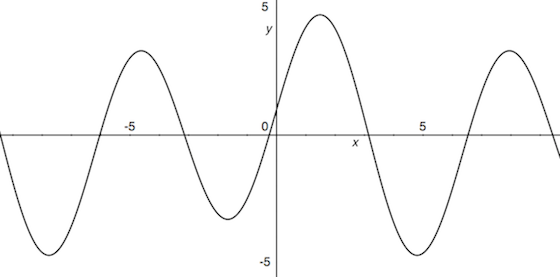#### Question 2 2. For these questions, I strongly recommend using either the CLEP calculator (found online) or one you use in class. Find x.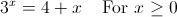#### Question 4 4. For these questions, I strongly recommend using either the CLEP calculator (found online) or one you use in class. At what is the minimum value of f(x) between 0 and 1?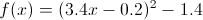#### Question 5 5. For these questions, I strongly recommend using either the CLEP calculator (found online) or one you use in class. Find x.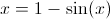### Page 2

#### Question 7 7. For these questions, I strongly recommend using either the CLEP calculator (found online) or one you use in class. Find x.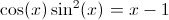#### Question 9 9. For these questions, I strongly recommend using either the CLEP calculator (found online) or one you use in class. What is the value of x where the following two lines intersect?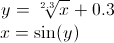### Page 3

#### Question 12 12. For these questions, I strongly recommend using either the CLEP calculator (found online) or one you use in class. Find x.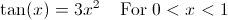### Page 4

#### Question 17 17. For these questions, I strongly recommend using either the CLEP calculator (found online) or one you use in class. Where is the maximum value of f(x) between 0 and pi?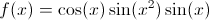#### Question 18 18. For these questions, I strongly recommend using either the CLEP calculator (found online) or one you use in class. Find x.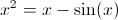#### Question 20 20. For these questions, I strongly recommend using either the CLEP calculator (found online) or one you use in class. What is the root of f(x)? (where f(x) is equal to zero)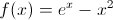#### Using a Scientific Calculator for Calculus Chapter Exam Instructions

Choose your answers to the questions and click 'Next' to see the next set of questions. You can skip questions if you would like and come back to them later with the yellow "Go To First Skipped Question" button. When you have completed the practice exam, a green submit button will appear. Click it to see your results. Good luck!

Support